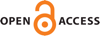Download this articleFor screen For printingRecent IssuesThe Journal About the Journal Editorial Board Editors’ Interests Subscriptions Submission Guidelines Submission Form Policies for Authors Ethics Statement ISSN: 1948-206X (e-only) ISSN: 2157-5045 (print) Author Index To Appear Other MSP JournalsLong time solutions for quasilinear Hamiltonian perturbations of Schrödinger and Klein–Gordon equations on tori

### Roberto Feola, Benoît Grébert and Felice Iandoli

Vol. 16 (2023), No. 5, 1133–1203##### Abstract

We consider quasilinear, Hamiltonian perturbations of the cubic Schrödinger and of the cubic (derivative) Klein–Gordon equations on the $d$-dimensional torus. If $𝜖\ll 1$ is the size of the initial datum, we prove that the lifespan of solutions is strictly larger than the local existence time ${𝜖}^{-2}$. More precisely, concerning the Schrödinger equation we show that the lifespan is at least of order $O\left({𝜖}^{-4}\right)$, and in the Klein–Gordon case we prove that the solutions exist at least for a time of order $O\left({𝜖}^{-{8∕3}^{-}}\right)$ as soon as $d\ge 3$. Regarding the Klein–Gordon equation, our result presents novelties also in the case of semilinear perturbations: we show that the lifespan is at least of order $O\left({𝜖}^{-{10∕3}^{-}}\right)$, improving, for cubic nonlinearities and $d\ge 4$, the general results of Delort (J. Anal. Math. 107 (2009), 161–194) and Fang and Zhang (J. Differential Equations 249:1 (2010), 151–179).

##### Keywords
quasilinear equations, paradifferential calculus, energy estimates, small divisors
##### Mathematical Subject Classification
Primary: 37K45, 35S50, 35B35, 35B45, 35L05, 35Q55
##### Milestones